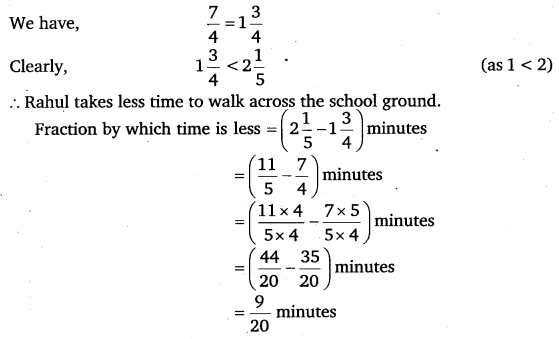# Class 6 Maths NCERT Solutions for Chapter 7 Fractions Ex – 7.6

## Fractions

Question 1.
Solve: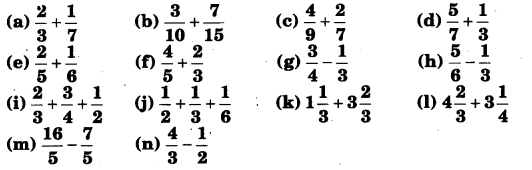Solution: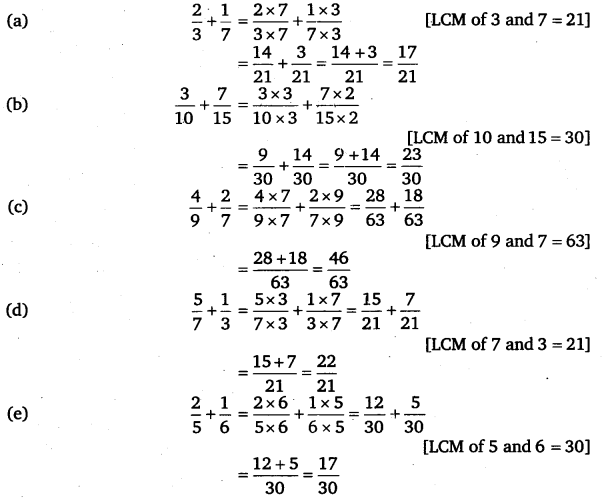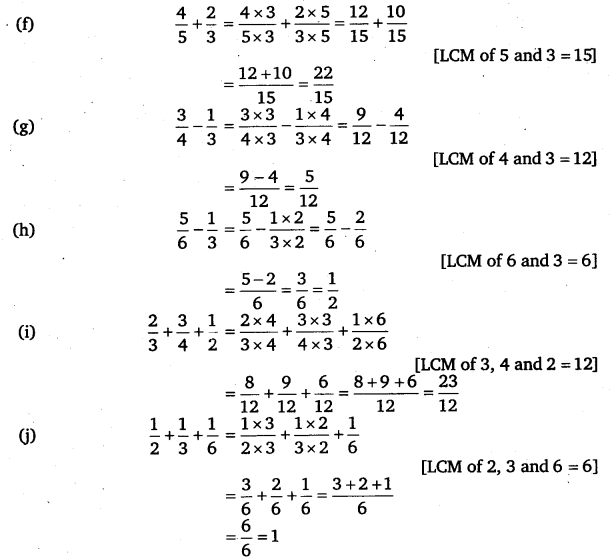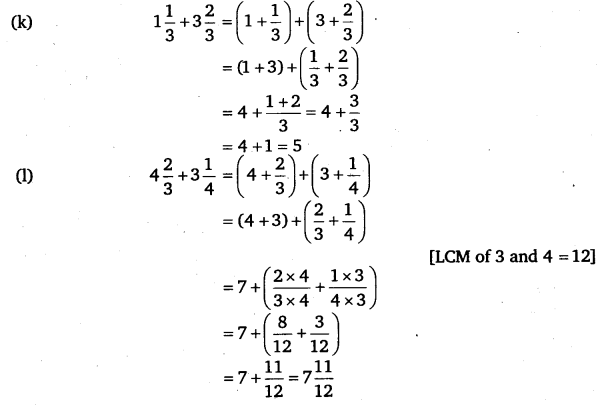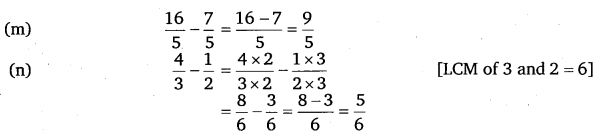Question 2.
Sarita bought$\frac { 2 }{ 5 }$ metre of ribbon and Lalita$\frac { 3 }{ 4 }$ metre of ribbon.
What is the total length of the ribbon they bought?

Solution:
Ribbon bought of Sarita =$\frac { 2 }{ 5 }$ m
Ribbon bought of Lalita =$\frac { 3 }{ 4 }$ m
Total length of the ribbon bought =$\left( \frac { 2 }{ 5 } +\frac { 3 }{ 4 } \right)$ m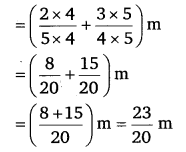Question 3.
Naina was given$1\frac { 1 }{ 2 }$ piece of cake and Najma was given$1\frac { 1 }{ 3 }$piece of cake. Find the total amount of cake was given to both of them.

Solution:
Number of pieces of cake consumed by Naina =$1\frac { 1 }{ 2 }$
Number of pieces of cake consumed by Najma =$1\frac { 1 }{ 3 }$
Total amount of cake consumed =$1\frac { 1 }{ 2 } +1\frac { 1 }{ 3 }$Question 4.
Fill in the boxes:Solution: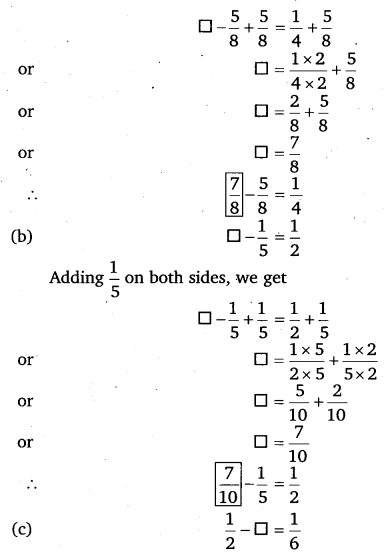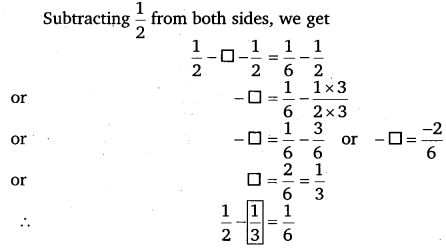Question 5.Solution: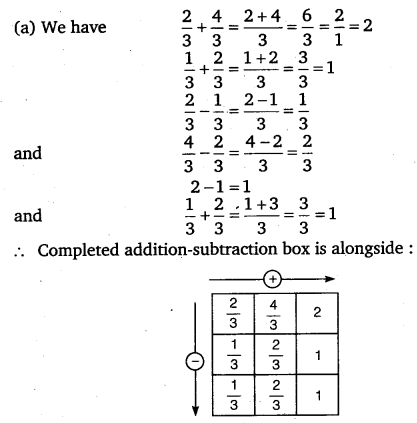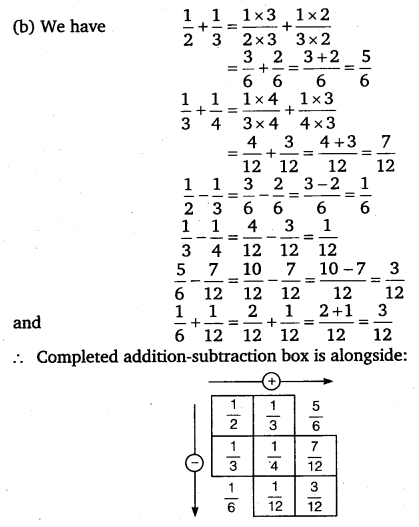Question 6.
A piece of wire$\frac { 7 }{ 8 }$ metre long broke into two pieces. One piece was$\frac { 1 }{ 4 }$ metre long. How long is the other piece?

Solution:
Total length of wire =$\frac { 7 }{ 8 }$ metre
Length of a piece of wire =$\frac { 1 }{ 4 }$ metre
∴ Other piece of wire =$\left( \frac { 7 }{ 8 } -\frac { 1 }{ 4 } \right)$ metreQuestion 7.
Nandini’s house is$\frac { 9 }{ 10 }$ km from her school. She walked some distance and then took a bus for$\frac { 1 }{ 2 }$ km to reach the school. How

Solution:
Total distance of Nandini’s house from her school =$\frac { 9 }{ 10 }$ km
Distance for which bus was taken =$\frac { 1 }{ 2 }$ km
∴ Distance walked by Nandini for home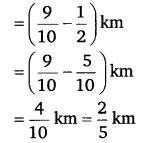Question 8.

Asha and Samuel have bookshelves of the same size partly filled with hooks. Asha’s shelf is$\frac { 2 }{ 5 }$ th full and Samuel’s shelf is$\frac { 5 }{ 6 }$ th full. Whose bookshelf is more full? By what fraction?

Solution: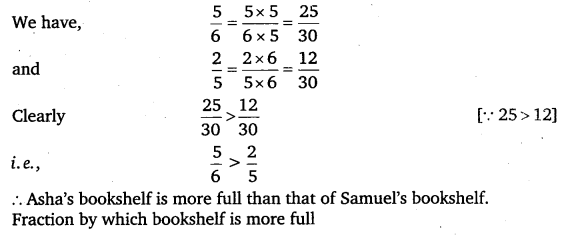Question 9.
Jaidev takes$2\frac { 1 }{ 5 }$ minutes to walk across the school ground. Rahul takes$\frac { 7 }{ 4 }$ minutes to do the same. Who takes less time and by what fraction?

Solution: